# The Model Student: A Knotty Problem, Part 1

The Model Student: A Knotty Problem, Part 1

By Richard Harris

Richard Harris explores more of the mathematics of modelling problems with computers.

If there's one thing that's guaranteed to irritate me, it's headphones. I don't mean the continual tinny noise pollution that thoughtless public transport patrons inevitably inflict upon their unfortunate fellow passengers; I'm far too busy inflicting my own tinny noise pollution on them to pay it any heed. No, I refer instead to their annoying tendency to tie themselves up in knots at every conceivable opportunity. As foolish as it is to anthropomorphise, I can't help but suspect that they are possessed of a demonic nature; that they will not rest until they have damned all of humanity to an eternal tortured state of minor inconvenience.

It's not just headphones either. If anything, fairy lights are even more belligerent. It seems that no matter how carefully I pack them away with the other Christmas decorations they will, 11 months or so later, have contrived to rearrange themselves into a tangle of Gordian complexity. Thus far I have resisted the temptation to assume the mantle of Alexander and take a pair of scissors to the blasted things; I suppose that it's only a matter of time before I succumb.

I am not alone in my frustration. Jerome K. Jerome [ Jerome89 ] made the same observation of tow-lines as long ago as 1889:

There may be tow-lines that are a credit to their profession - conscientious, respectable tow-lines - tow-lines that do not imagine that they are crotchet-work, and try to knit themselves up into antimacassars the instant they are left to themselves. I say there may be such tow-lines; I sincerely hope there are. But I have not met with them.

So are I and my illustrious forebears suffering from an overactive imagination or do we really live in a universe in which strings and cables spontaneously tie themselves into knots?

Believe it or not, there is an entire field of mathematics dedicated to the study and classification of knots that does, in fact, have something to say on the matter (Brian Hayes [ Hayes ] has an interesting discussion on the subject of random closed knots). Unfortunately, it's an extremely difficult subject. So much so that even determining whether or not two knots are equivalent is still an unsolved problem.

Whilst knot theory would certainly shed a great deal of light upon the subject, I'm afraid I might sprain something trying to understand it. Instead, I shall propose a simpler model which, with luck, won't cause me any permanent injury.

That model is a random walk.

Generally speaking, a random walk is a sequence generated by a series of random steps from some given starting position. In this case, we will model a string as a chain of finitely sized inflexible links. We can divide the two dimensional surface onto which we lower it, a table for example, into a discrete grid. We can then hypothesise that each link will occupy the location of the previous link or of one of its eight neighbours with equal probability, as illustrated in figure 1 (which shows the candidate steps in a two dimensional random walk). Figure 2 illustrates one such walk (a 9-step random walk).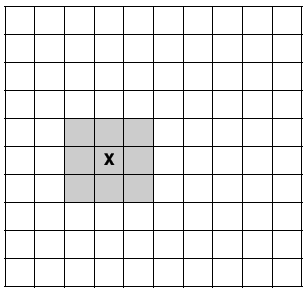Figure 1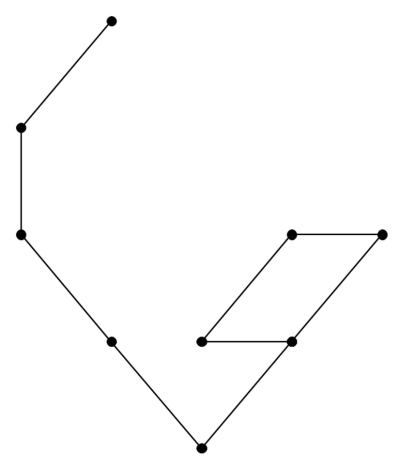Figure 2

The properties of random walks have been studied for a great many years since they are, in the continuous limit, the mathematical model for Brownian motion. This is the random motion of tiny particles suspended in liquid or gas named after Robert Brown who observed it for pollen grains floating in water in 1827 [ Brown28 ]. Some fifty years later, the mathematics of Brownian motion was described by Thorvald Thiele [ Thiele80 ]. Since then it has been a subject of great interest to mathematicians and physicists, and more recently financiers. The latter has grown into a lucrative field since there are strong theoretical arguments that share prices exhibit a form of Brownian motion [ Bachelier00 , Hull05 ].

The relationship between random walks and knots has not escaped the attention of mathematicians. Nechaev [ Nechaev98 ] provides a thorough review of the current state of the art, a state which I must admit is sufficiently advanced that I am reticent to tackle it.

So how should an amateur like me relate random walks to the question at hand? Well, each time the cable crosses itself it is presented with an opportunity to tangle. In my experience cables are loathe to pass up such opportunities lightly, so the number of self crossings should hopefully give some insight into their tendency to become knotted.

The obvious way to measure the number of self crossings is to generate every possible walk of a given length and simply count the number of times each of them crossed itself, or other words returns to a point it has already visited. Equally obvious is that this will be extremely computationally expensive. Since each step has nine possible outcomes, a walk of n steps will have a total of 9 n distinct outcomes.

Nevertheless, it's worth pursuing since it leads to some interesting C++.

Before we start we'll need some classes to manage the random walks and keep track of the number of crossings. Let's start with a class to represent a position on the planar grid. Listing 1 shows a class to represent a position in a random walk.

 ``` namespace knots { class position { public: position(); position(long x, long y); position move(long dx, long dy) const; bool operator<(const position &rhs) const; bool operator==(const position &rhs) const; private: long x_; long y_; }; } ``` Listing 1

The important features of this class are that it supports moving from one position to another with the move member function and that it defines a strict weak ordering with operator< , making it possible to use it as a key in a std::set or std::map .

Their implementation is fairly simple, the only trap being ensuring that operator< is a strict weak ordering. We can ensure this by making it a lexicographical comparison. Note that this has no real mathematical meaning and serves only to make position compatible with associative containers. Listing 2 shows an implementation of move and comparison.

 ``` knots::position knots::position::move(long dx, long dy) const { return position(x_+dx, y_+dy); } bool knots::position::operator<( const position &rhs) const { return x_

Once again, we'll need a histogram to keep track of the number of self crossings of the random walks. The histogram shall assume that every self crossing results in a knot, thus measuring the worst possible outcome. Apart from some of the names, this is pretty much identical to the one we used for the travelling salesman problem.

Listing 3 shows a class to maintain a histogram of knots. Again, most of the member functions are straightforward. The constructors are amongst those that require some care. Listing 4 shows construction of the knot histogram.

 ``` namespace knots { class knot_histogram { public: struct value_type { double knots; size_t count; value_type(); value_type(double k, size_t c); }; typedef std::vector histogram_type; typedef histogram_type::const_iterator const_iterator; typedef const value_type & const_reference; typedef histogram_type::size_type size_type; knot_histogram(); explicit knot_histogram(size_t length); knot_histogram(size_type buckets, size_t knots_per_bucket); bool empty() const; size_type size() const; size_type walk_length() const; const_iterator begin() const; const_iterator end() const; const_reference operator[](size_type i) const; const_reference at(size_type i) const; void add(size_t knots); private: void init(); size_type knots_per_bucket_; histogram_type histogram_; }; } ``` Listing 3

 ``` knots::knot_histogram::knot_histogram() { } knots::knot_histogram::knot_histogram( size_t length) : knots_per_bucket_(1), histogram_(length) { init(); } knots::knot_histogram::knot_histogram( size_type buckets, size_t knots_per_bucket) : knots_per_bucket_(knots_per_bucket), histogram_(buckets) { init(); } ``` Listing 4

By default we use one bucket per step of the walk since we know that the maximum possible number of self crossings results from every step staying in the same cell. As before, the init member function initialises the buckets (Listing 5).

 ``` void knots::knot_histogram::init() { if(empty()) throw std::invalid_argument(""); histogram_type::iterator first = histogram_.begin(); histogram_type::iterator last = histogram_.end(); size_t knots = 0; --last; while(first!=last) { knots += knots_per_bucket_; *first++ = value_type( double(knots)/double(walk_length()), 0); } *first = value_type(1.0, 0); } ``` Listing 5

This time it's slightly simpler since we've forced the user to pass in the number of knots per bucket in the histogram. Note that we're using the proportion of the walk that's knotted, rather than the absolute number of knots, as the value for the bucket. The value represents the upper bound for the bucket in question, with the lower bound being the value of the previous bucket, or zero for the first.

Once again we'll be exploiting the fact that the buckets are evenly distributed over the unit range to simplify adding a knot count to the histogram. We need only divide the number of knots by the knots per bucket to identify the correct bucket.

If we were to do this alone, however, we would have a problem with the walk in which each of the n steps is to remain in the same location. This would have n crossings which would lead us to attempt to access the bucket after the last in the histogram. We therefore make a slight approximation and ignore that walk. Naturally this introduces an error, but since it is but one walk out of 9 n it shouldn't have a significant impact on the results.

Listing 6 shows adding a walk to the histogram. Note that we can recover the length of the walk by simply multiplying the number of buckets by the number of knots per bucket:

```      knots::knot_histogram::size_type
knots::knot_histogram::walk_length() const
{
return size() * knots_per_bucket_;
}

```
 ``` void knots::knot_histogram::add(size_t knots) { knots /= knots_per_bucket_; if(knots

Finally, we need a way to represent a random walk and to count the number of self crossings. Observing that each step has nine possible outcomes of equal probability, we can trivially represent a walk with a std::vector<unsigned char> element of which contains a value in the range zero up to and including eight.

In addition to a typedef formalising this definition of a random walk, the following includes the declaration for a function to count the number of crossings:

```      namespace knots
{
typedef std::vector<unsigned char> walk;
size_t crossings(const walk &w);
}

```

The implementation of crossings needs to iterate through each position in the walk and check whether or not it has already been visited. Since we went out of our way to make position compatible with std::set , it seems to be a natural choice to keep track of the visited position s.

Listing 7 shows counting the self crossings.

 ``` size_t knots::crossings(const walk &w) { size_t knots = 0; position p(0, 0); std::set visited; walk::const_iterator first = w.begin(); walk::const_iterator last = w.end(); visited.insert(p); while(first!=last) { if(*first>8) throw std::invalid_argument(""); long dx = long(*first)%3 - 1; long dy = long(*first)/3 - 1; p = p.move(dx, dy); if(!visited.insert(p).second) ++knots; ++first; } return knots; } ``` Listing 7

The principal trick we're exploiting to update the position as we iterate through the walk is the use of integer division and modulus to generate the nine distinct steps. At the risk of labouring the point, figure 3 (mapping the step id to the offsets) illustrates how this works.

step step%3-1 step/3-1
0 -1 -1
1 0 -1
2 1 -1
3 -1 0
4 0 0
5 1 0
6 -1 1
7 0 1
8 1 1
Figure 3

Ideally, instead of throwing an exception when a step in a walk is too large we should create a class to represent a limited range integer and use it instead of unsigned char . That would be a little too much work for this article however, so I'm not going to bother.

So now we're ready to start generating random walks and taking some measurements. The temptation to use a recursive algorithm to generate the full set of walks of a given length is strong; the approach is a natural fit for this kind of problem. At each step we can iterate over every possible move and then recursively generate the remainder of the walk. Each time we reach the end of a walk we add the number of crossings to the histogram to record the results. Listing 8, which calculates the histogram of self crossings, illustrates how we might implement this.

 ``` void knots::full_crossings( walk &w, knot_histogram &h, size_t n) { if(n==w.size()) { h.add(crossings(w)); } else { for(size_t i=0;i!=9;++i) { w[n] = i; full_crossings(w, h, n+1); } } } void knots::full_crossings(knot_histogram &h) { walk w(h.walk_length()); full_crossings(w, h, 0); } ``` Listing 8

Whilst recursion can greatly simplify the expression of these kinds of operations, it can, in some situations at least, be less efficient than iteration.

Is it possible to express this operation succinctly with an iterative approach?

Well, std::next_permutation provides us with a clue as to how to go about it. It takes an iterator range and transforms it to the lexicographically next greatest permutation of the contained values. What we need is a next_state function that transforms the values in an iterator range to the lexicographically next greatest set of values.

To make it as general as possible, we shouldn't assume that the values are integer types. We must assume a method for transforming a value to the next greatest, however. We have a likely candidate in operator++ ; it already does what we need for integer types and can be overloaded for user defined classes. If overloading is not appropriate, perhaps the state transformation is too computationally expensive or our state is formed from classes that we cannot modify, we could instead use iterators into a container of states.

So how should the algorithm operate? Well, we need simply observe that iterating through a set of states is equivalent to counting through a set of integers. Each time a digit takes its maximum value, or upper bound, we set it to its minimum value, or lower bound, and perform a carry; i.e. increment the next most significant digit.

We begin with a helper function to increment a digit and indicate whether or not we need to carry. Listing 9 shows rotating a digit of the state.

 ``` template bool rotate_state(BidIt it, const T &lb, const T &ub) { bool last = ++*it==ub; if(last) *it=lb; return last; } ``` Listing 9

Given this we need only take care that the carry operation ripples through the iterator range correctly. Listing 10 shows generating the next state.

 ``` template bool next_state(BidIt first, BidIt last, const T &ub, const T &lb = T()) BidIt it = last; while(it!=first && rotate_state(--it, lb, ub)); return first!=last && (it!=first || *it!=lb); } ``` Listing 10

All of the work is done in the while statement. We iterate backwards through the range, rotating the state for as long as we need to carry. If we have reached the first digit and it has been rotated back to the lower bound, we have reached the state in which every digit has the minimum value. Assuming that this was the starting state, have exhausted all possible states and return false to indicate this.

Note that we pass in the lower and upper bounds in decreasing order. This is because there is a reasonable value for the lower bound, namely the default constructed value. Note that built in types will be zero initialised which is very likely to be what we want for integer valued states.

Listing 11 illustrates the use of next_state for states with integer valued digits and Figure 4 illustrates the output from this code snippet.

 ``` std::vector state(3, 0); std::ostream_iterator out(std::cout); do { std::copy(state.begin(), state.end(), out); std::cout << std::endl; } while(next_state(state.begin(), state.end(), 2)); ``` Listing 11

 ``` 000 001 010 011 100 101 110 111 ``` Figure 4

Listing 12 illustrates the more complex task of iterating through states with a compound type for its digits, in this case std::string . As discussed earlier, if we store the set of digits in a container we can use iterators to represent the current value of a digit. The output of Listing 12 is shown in Figure 5.

 ``` typedef std::vector digits_type; typedef std::vector< digits_type::const_iterator> state_type; digits_type digits(3); digits = " "; digits = "o"; digits = "x"; state_type state(2, digits.begin()); std::cout << "12" << std::endl; do { state_type::const_iterator first = state.begin(); state_type::const_iterator last = state.end(); while(first!=last) std::cout << **first++; std::cout << std::endl; } while(next_state(state.begin(), state.end(), digits.end(), digits.begin())); ``` Listing 12

 ``` 12 o x o oo ox x xo xx ``` Figure 5

As can be seen, the complexity arises from setting up the digits and printing out the states rather than from iterating through them, which remains as simple as it was in the integer case.

Rewriting full_crossings to exploit next_state is relatively straightforward. If anything, it's even simpler now than it was with a recursive implementation.

Listing 13 shows calculating the histogram of self crossings.

 ``` void knots::full_crossings(knot_histogram &h) { if(h.walk_length()) { walk w(h.walk_length(), 0); h.add(crossings(w)); while(next_state(w.begin(), w.end(), 9)) { h.add(crossings(w)); } } } ``` Listing 13

But is it any more efficient?

Sadly, not very much so, as Figure 6, which shows the computational expense of generating knot histograms, clearly illustrates.

n recursed time (seconds) iterated time (seconds)
5 0.17 0.17
6 1.80 1.69
7 17.80 16.89
8 175.56 167.51
9 1701.56 1650.41
Figure 6

So why not? Could it be that the cost of counting the number of self crossings vastly outweighs the cost of recursively constructing the walk; the use of std::set to keep track of the visited locations results in O( n ln n ) complexity for n crossings. Or perhaps that my compiler and the hardware I'm using are able to mitigate the cost of recursion that I recall from my youth.

Actually, no.

Had I profiled the code in the first place as did one of the reviewers (thanks Roger), I'd have noticed that most of the time is spent inserting and erasing elements from std::set . Unfortunately I've not given myself enough time to replace std::set with something more efficient.

However, I shall continue to use the iterative approach because of the surprising fact that it actually simplifies the code.

So what do the histograms look like? Figure 7 shows the results for exhaustive enumeration of walks of lengths from six to nine steps. Before looking at them, it's worth pointing out again that our histogram records the number of knots as a proportion of the length of the walk, so the histograms will record counts from 0.0 to 1.0.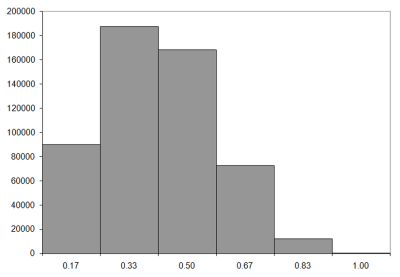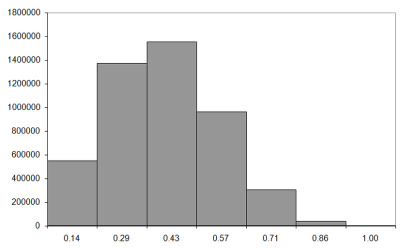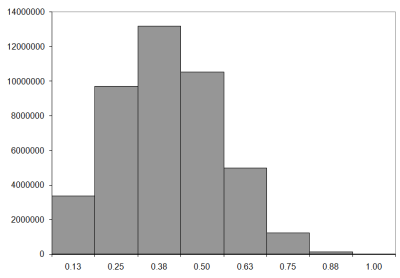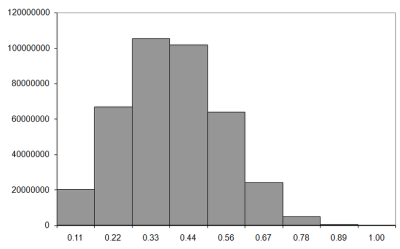Figure 7

So how are the knots distributed? Is there a standard statistical distribution that describes them? Well, I have my suspicions, but before we can check them we need to know the average, or mean, number of knots for a walk of a given length.

We can calculate the observed mean number of crossings directly from these histograms. Firstly we should note that the walks recorded in each bucket are those with lengths less than the label, but greater than or equal to that of the previous bucket. For these short walks, we have one knot count per bucket, so the number of knots must be the previous bucket's value and zero for the first bucket.

To calculate the mean, we simply add up the proportion of the walks in each bucket multiplied by the number of knots it represents. Recall that the mean is defined as the expected number of knots and that we use E(x) to denote the expected value of x .

```         E(knots)   n i-1   count(i)
———————— = ∑ ——— × ————————
n      i=1  n     9n-1  ```

As pointed out above, we're ignoring the walk with the maximum number of knots, so there are only 9 n -1 walks.

The results of this calculation are given in Figure 8, which shows the expected proportional number of knots for walks of length 6, 7, 8 and 9.

n E(knots)/n
6 0.2492
7 0.2623
8 0.2737
9 0.2837
Figure 8

So can we deduce an explicit, or closed form, formula for the expected number of knots? Well, we shall have a go at it next time.

## Acknowledgements

[ Bachelier00] Bachelier, L., Théorie de la Spéculation , PhD thesis, 1900.

[ Brown28] Brown, R., 'A brief account of microscopical observations made in the months of June, July and August, 1827, on the particles contained in the pollen of plants; and on the general existence of active molecules in organic and inorganic bodies', Edinburgh New Philosophical Journal , July-September, pp. 358-371, 1828.

[ Feller68] Feller, W., An Introduction to Probability Theory and its Applications, vol. 1, 3rd ed., Wiley, 1968.

[ Hayes97] Hayes, B., 'Square Knots', American Scientist , vol. 85, num. 6, pp. 506-510, 1997

[ Hull05] Hull, J., Options, Futures and Other Derivatives , 6th ed., Prentice Hall, 2005.

[ Jerome89] Jerome, J. K., Three Men in a Boat , J. W. Arrowsmith, 1889.

[ Nechaev98] Nechaev, S., 'Statistics of Knots and Entangled Random Walks', arXiv:cond-mat/9812205 v1, www.arxiv.org, 1998.

[ Press92] Press et al., Numerical Recipes in C , 2nd ed., Cambridge, 1992

[ Robbins55] Robbins, H., 'A Remark on Stirling's Formula', American Mathematical Monthly , vol. 62, pp. 26-29, 1955.

[ Thiele80] Theile, T. N., 'Om Anvendelse af mindste Kvadraters Methode i nogle Tilfælde, hvor en Komplikation af visse Slags uensartede tilfældige Fejlkilder giver Fejlene en 'systematisk' Karakter', Vidensk. Selsk. Skr. 5. Rk., naturvid. og mat. Afd. , vol. 12, pp. 381-408, 1880.